## 2.13  Nonsingular Generalized Coordinates

The Euler angles provide a convenient way to parameterize the orientation of a rigid body. However, the equations of motion derived for them have singularities. Though we can avoid the singularities by using other Euler-like combinations with different singularities, this kludge is not very satisfying. Let's brainstorm a bit and see if we can come up with something better.

What does it take to specify an orientation? Perhaps we can take a hint from Euler's theorem. Recall that Euler's theorem states that any orientation can be reached with a single rotation. So one idea to specify the orientation of a body is to parameterize this single rotation that does the job. To specify this rotation we need to specify the rotation axis and the amount of rotation. We contrast this with the Euler angles, which specify three successive rotations. These three rotations need not have any relation to the single composite rotation that gives the orientation. Isn't it curious that the Euler angles make no use of Euler's theorem?

We can think of several ways of specifying a rotation. One way would be to specify the rotation axis by the latitude and the longitude at which the rotation axis pierces a sphere. The amount of rotation needed to take the body from the reference position could be specified by one more angle. We can predict, though, that this choice of coordinates will have similar problems to those of the Euler angles: if the amount of rotation is zero, then the latitude and longitude of the rotation axis are undefined. So the Lagrange equations for these angles are probably singular. Another idea, without this defect, is to represent the rotation by the rectangular components of an orientation vector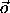; we take the direction of the orientation vector to be the same as the axis of rotation that takes the body from the reference orientation to the present orientation, and the length of the orientation vector is the angle by which the body must be rotated, in a right-hand sense, about the orientation vector. With this choice of coordinates, if the angle of rotation is zero then the length of the vector is zero and has no unwanted direction. This choice looks promising.

We denote the rectangular components ofby the following: ( ox, oy, oz ); these will be our generalized coordinates. The magnitude o = (ox2 + oy2 + oz2)1/2 is the angle of rotation. The axis of the rotation is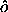=/ o. We denote the components ofbyx,y, andz. The first step in implementing the components of the orientation vector as generalized coordinates is to construct the rotation M to which the orientation vectorcorresponds. Let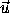' be a vector to one of the constituents of the body in the reference orientation, andbe the vector to that constituent after rotation by M: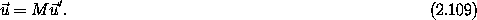We can determine M by considering how the rotation represented byaffects the vector'. The component of' parallel tois unaffected. The perpendicular component is reduced by the cosine of the rotation angle, and a component perpendicular to these two is generated that is proportional to the sine of the rotation angle and the magnitude of the perpendicular component. Let (')|| = (' ·)and (')| =' - (')||; then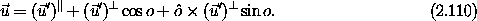From this expression we can construct the equivalent rotation matrix. First define some useful primitive matrices: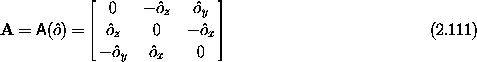and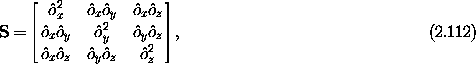with the identity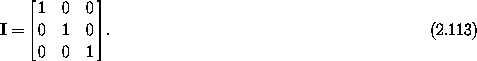The matrix A is antisymmetric and S is the symmetric outer product of the components of. The matrix A implements the cross product ofwith other vectors, and the matrix S projects vectors to the orientation vector. We have the following identities: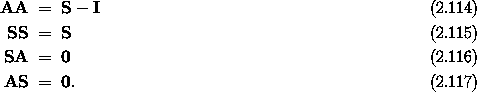In terms of these matrices, the rotation matrix is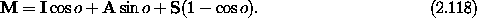The inverse of a rotation is a rotation about the same axis but by the negative of the rotation angle. Thus the inverse of M can be written down immediately: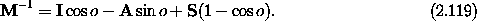We verify that the inverse of the rotation matrix is the transpose of the rotation matrix by recalling that I and S are symmetric and A is antisymmetric.

The computation of the angular velocity vector from MT and DM is straightforward, though tedious; the angular velocity vector turns out to have a simple form: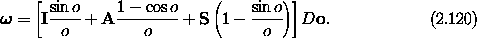The components of the angular velocity vector on the principal axes can be found by multiplying the above by M-1 = MT: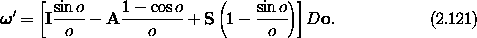Let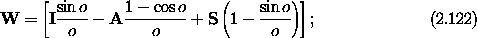then we have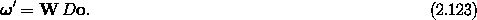Solving, we find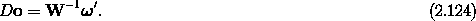The matrix W is not an orthogonal matrix, so its inverse is not trivial, but we can use the properties of the primitive matrices to find it. Suppose we have a matrix of the form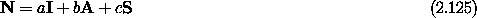that we wish to invert. Let's guess that the inverse matrix has a similar form: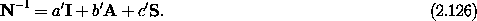We wish to find the coefficients a', b', and c' so that NN-1 = I. We find three conditions on the coefficients: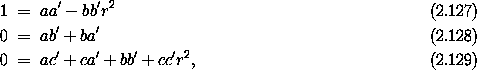with solution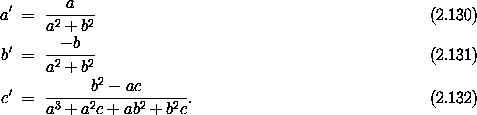We can now invert the matrix W, using its representation in terms of primitive matrices, to find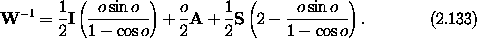Note that all terms have finite limits as o --> 0. There is, however, a new singularity. As o --> 2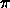two of the denominators become singular, but there the zeros in the numerators are not strong enough to kill the singularity. This is the expected singularity that corresponds to the fact that at radius 2the orientation vector corresponds to no rotation, but nevertheless specifies a rotation axis. This singularity is easy to avoid: whenever the orientation vector develops a magnitude larger than, simply replace it by the equivalent orientation vector- 2.

We can write the equations governing the evolution of the orientation as a vector equation in terms of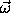' = M-1: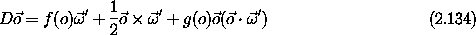with two auxiliary functions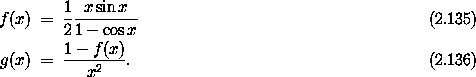The equation of motion for the orientation vector is surprisingly simple. Both auxiliary functions have finite limits at zero: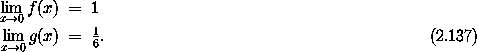Orientation vectors with magnitude less than or equal toare enough to specify all orientations, and the equations of motion in this region have no singularities. The orientation vector may develop magnitudes greater than, but then we replace it by the equivalent orientation vector with magnitude less than. And there is no hurry to do this because the equations are not singular until the magnitude reaches 2. Thus we have a complete nonsingular specification of the rigid body dynamics.

#### A practical matter

In order to use the orientation vector, we are presented with the practical problem of converting between the orientation vector representation of the orientation and other representations. We can consider the rotation matrix M as an intermediate universal representation. Whatever generalized coordinates have been chosen, we must be able to compute the rotation matrix to which the coordinates correspond. We must also solve the converse problem -- the determination of the generalized coordinates from the rotation matrix.

We already have the explicit form for the rotation matrix in terms of the orientation vector in equation (2.118), repeated here for convenience: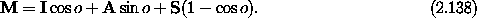We can solve the converse problem by examining this same equation. We note that of the contributions to M two parts are symmetric and one is antisymmetric. We can isolate the antisymmetric component by subtracting the transpose. We have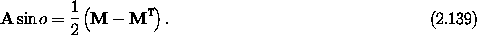But the matrix A is simply related to the orientation vector: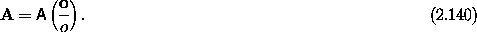We use the inverse operation A-1 that extracts the components of a 3-vector from an antisymmetric 3 × 3 matrix. So we have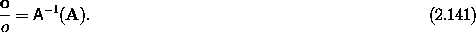Note that information about the magnitude o of the rotation is not available in A by itself. However, the combination of M and its transpose produces a scaled version of A from which the magnitude of the rotation can be recovered: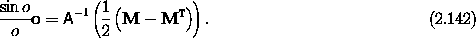The length of the vector represented by the components on the left-hand side is just sin o. This does not determine o uniquely, because o spans the interval 0 to. To completely determine o and thuswe need more information, say by determining cos o.

We can get cos o easily enough. Examination of the components shows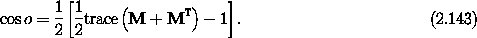Having determined both the sine and the cosine of o, we can determine o. Some of these expressions contain divisions by o that may be zero, but if o = 0 then the orientation vector is just the zero vector. This completes the solution of the practical problem of going to and from the orientation vector.

#### Composition of rotations

We can ask the following question: ``To which rotation does the composition of two rotations correspond?'' Or alternatively, ``What is the algebra of orientation vectors?'' We have all the pieces; answering this question is just a matter of computation. Given two rotations represented by the rotation matrices M1 and M2, the rotation matrix of the composition of these rotations is M = M2 M1. Each of these rotation matrices can be converted to the equivalent orientation vector. We can define the composition=2 o1.

Let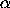= (sin o) / o, ß = (1 - cos o)/o2, and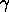= cos o. By direct calculation we find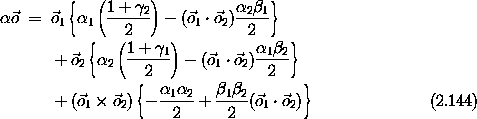and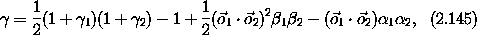which together determine.

Well, the formulas are rather complicated, but it turns out that with a little rearrangement they can be made quite simple. Let c = cos ( o/2 ) and s = sin ( o/2), and define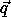= (s / o). The vectoris a scaled version of; instead of having the magnitude o asdoes, the vectorhas the magnitude s = sin (o/2). Notice that if o is restricted to magnitudes less thanthen the magnitude of the rotation o can be recovered from the magnitude of. Thus, with this restriction, the vectorcorresponds to a unique rotation, and no extra information is needed. Nevertheless, it is convenient to keep track of the cosine of the half-angle as well as the sine; so let q = c = cos ( o/2).22 The magnitude of= s and q = c, so q2 +·= 1. We can reexpress the formulas for the composition of two rotations in terms ofand q for each rotation. We have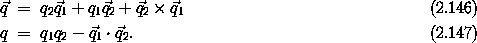Now that is a significant simplification! The 4-tuple formed from q with the three components ofis the tuple of components of Hamilton's quaternion. We see that the vector part of the quaternion that represents an orientation is a scaled version of the orientation vector.

Hamilton discovered an even more elegant way of writing the formula for the composition of two rotations. Introduce three unit quaternions i, j, k, such that i2 = j2 = k2 = - 1, ij = k, jk = i, ki = j, and each pair of distinct unit quaternions anticommute: ij = - ji, and so on. Denote the three components ofby ( q1, q2, q3 ), and q by q0. Then define the composite quaternion q = q0 + i q1 + j q2 + k q3. With the rule for how the unit quaternions multiply, the formula for the composition of two rotations becomes simply a multiplication. The quaternions generalize the idea of complex numbers. In fact, they are the only algebraically closed field besides complex numbers. The unit quaternions cannot be represented simply as real numbers or complex numbers, particularly because of their anticommuting properties. However, they do have a representation as 2 × 2 matrices of complex numbers. The units are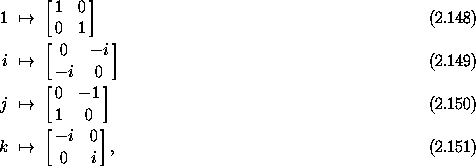where the i on the right-hand side is the imaginary unit i2 = - 1. These matrices are related to the Pauli spin matrices. There are other representations, but further discussion of representations of rotations would carry us too far afield.

If we are faced with the task of composing rotations repeatedly, then the quaternions will be a handy intermediate representation. The quaternions also have the advantage that we do not need to worry about whether the angle of rotation is in the appropriate range. However, the equation of motion for the orientation vector is simpler than the equation of motion for the quaternion, so we will stick with the orientation vector when we need nonsingular equations of motion for the orientation.

Exercise 2.18.  Composition
Verify that the rule for composition of two rotations in terms of the orientation vectors (equations 2.144 and 2.144) is equivalent to the rule for multiplying two quaternions (equation 2.147).

Exercise 2.19.  Equation of motion
Find the equation of motion for the orientation quaternion in terms of the angular velocity vector.

22 This notation has the potential for great confusion: q is not the magnitude of the vector. Watch out!